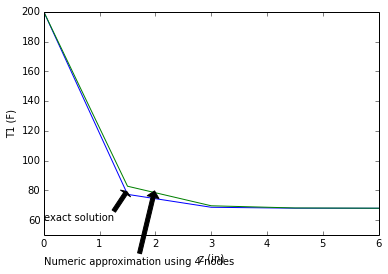# Chapter 3:Steady-State Conduction in Multiple Dimensions¶

### Example 3.1 Page No.132¶

In :
OD=0.02858              # outer diameter in m
M=8.0                     # total number of heat-flow lanes
N=6.0                     # number of squares per lane
S_L=M/N                   # conduction shape factor
k=0.128          # thermal conductivity in W/(m.K) for concrete from appendix table B3
T1=85            # temperature of tube surface
T2=0             # temperature of ground beneath the slab

q_half=k*S_L*(T1-T2)
q=2*q_half

print"The total heat flow per tube is",round(q,0)," W/m"

The total heat flow per tube is 29.0  W/m


### Example 3.2 Page No.134¶

In :
import math
OD=10.74/12 # diameter in ft
R=OD/2
T1=140.0
T2=65.0
k=0.072 # thermal conductivity in BTU/(hr-ft. degree R)
d=18.0/12.0 # distance from centre-line
S_L=(2*math.pi)/(math.acosh(d/R))
q_L=k*S_L*(T1-T2)
print"The heat transferred from the buried pipe per unit length is ",round(q_L,2),"BTU/(hr.ft)"

The heat transferred from the buried pipe per unit length is  18.05 BTU/(hr.ft)


### Example 3.3 Page No.135¶

In :
k = 1.07           # thermal conductivity of silica brick from appendix table B3 in W/(m.K)
S1_A=0.138*0.138/0.006
nA=2

St_A=nA*S1_A      # Total shape factor of component A

S1_B=0.138*0.188/0.006
nB=4
St_B=nB*S1_B # Total shape factor of component B

S3_C=0.15*0.006
nC=8
St_C=nC*S3_C # Total shape factor of component C

S2_D=0.54*0.188
nD=4
St_D=nD*S2_D # Total shape factor of component D

S2_E=0.138*0.54
nE=8
St_E=nE*S2_E # Total shape factor of component E

S=St_A+St_B+St_C+St_D+St_E
T1=550
T2=30
q=k*S*(T1-T2)
S=St_A+St_B
q_1=k*S*(T1-T2)
Error=(q-q_1)/q

print"(a)The heat transferred through the walls of the furnace is ",round(q/1000,1),"kw"
print"(b The heat transferred is",q_1/1000,1,"kw"
print"  The error introduced by neglecting heat flow through the edges and corners is ",round(Error*100,1)," percent"

(a)The heat transferred through the walls of the furnace is  13.7 kw
(b The heat transferred is 13.1555216 1 kw
The error introduced by neglecting heat flow through the edges and corners is  4.1  percent


### Example 3.4 Page No. 142¶

In :
OD=4.5/12.0          # diameter in ft
R=OD/2.0

import math
L_A=4.5           # length in ft
S_A=(2*math.pi*L_A)/(math.log(2*(L_A)/R))
L_B=18 # length in ft
S_B=(2*math.pi*L_B)/(math.acosh(L_A/R))
S=2*S_A+S_B

print"The total conduction shape factor for the system is",round(S,1)

The total conduction shape factor for the system is 43.8


### Example 3.5 Page No. 151¶

In [ ]:
%matplotlib inline

In :
h=1.1           # convective coefficient in BTU/(hr.ft^2. degree R)
Tw=200.0
T_inf=68.0      # ambient temperature
k=0.47          # thermal conductivity in BTU/(hr.ft.degree R) from table B3
D=0.25/12       # diameter in ft

A=math.pi*D**(2)/4.0     # cross sectional area in ft^2
P=math.pi*D         # perimeter in ft
L=6/12.0 # length in ft
mL=L*((h*P)/(k*A))**(0.5)
dz=1.0
L=4.0
de=dz/L
K=2+(mL*de)**2

T4=T_inf+(Tw-T_inf)*(2/(K**4-4*K**2+2))
T3=T_inf+(Tw-T_inf)*(K/(K**4-4*K**2+2))
T2=T_inf+(Tw-T_inf)*((K**2-1)/(K**4-4*K**2+2))
T1=T_inf+(Tw-T_inf)*((K**3-3*K)/(K**4-4*K**2+2))

print"The temprature distribution is T4=",round(T4,2),"F"
print"The temprature distribution is T3=",round(T3,2),"F"
print"The temprature distribution is T2=",round(T2,2),"F"
print"The temprature distribution is T1=",round(T1,2),"F"

import matplotlib.pyplot as plt
import numpy as np
T=[200.0,77.33,68.66,68.05,68]
z=[0,1.5,3,4.5,6]
xlim(0,6)
ylim(50,200)
plt.ylabel('T (C)')
plt.xlabel('z (in)')
plt.annotate('Numeric approximation using 4 nodes', xy=(2,82), xytext=(0,30),
arrowprops=dict(facecolor='black', shrink=0.05),
)
a=plot(z,T)

T1=[200.0,82.81,69.66,68.19,68.04]
plt.ylabel('T1 (F)')
plt.xlabel('z (in)')
plt.annotate('exact solution', xy=(1.5,80), xytext=(0,60),
arrowprops=dict(facecolor='black', shrink=0.05),
)
a=plot(z,T1)
show(a)

The temprature distribution is T4= 68.04 F
The temprature distribution is T3= 68.19 F
The temprature distribution is T2= 69.68 F
The temprature distribution is T1= 82.82 FIn [ ]: